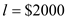## Quiz 18 : Externalities and Public GoodsLooking for Economics Homework Help?# Quiz 18 : Externalities and Public Goods

a) A firm in a perfectly competitive market is a price taker. Hence, it maximizes it profits by producing at a point at which marginal cost is equal to the prevailing price. As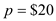and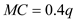For maximizing profit, firm will equate the price and the marginal cost. We get,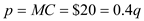From this we get the optimal quantity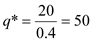b) The social optimum is given at the point where social marginal cost is equal to the social marginal benefit. In the case of a perfectly competitive firm, the social marginal benefit would be equal to the price. The social marginal cost however would be greater than the private marginal cost of the firm. The social marginal cost is given by 0.5q. So for social optimum output, the following should hold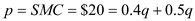From this we get the socially optimal quantity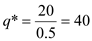The government can put a tax on each unit produced to get the firm to produce the socially optimal quantity while maximizing its profit. Let such a tax be t. Then the following should hold. For maximizing profit, firm will equate the price and the marginal cost. We get,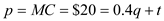Since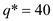Then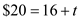This gives us the excise tax that the government should charge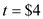c) The above results can also be shown in the graph below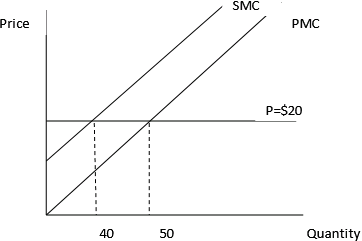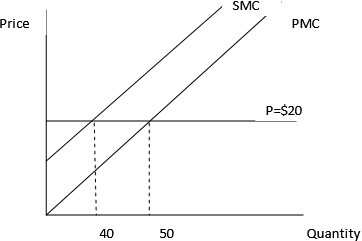a) Since there are 2 lakes, each angler while deciding which lake to fish in compares the average catch of the lakes. For equilibrium, it must be true that the average catch of both the lakes be equal otherwise an angler will have the incentive to switch to the lake offering higher average catch. Let l x be the number of anglers working on lake x. Hence, at equilibrium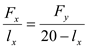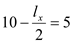So,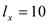As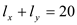...... (1) So,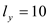Total number of fish caught is given by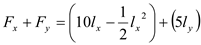...... (2) Substituting the values we get,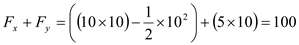b) The total number of fish caught is given by equation (2) reproduced belowWe maximize the profit function below. The constraint is given by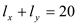His budget constraint is: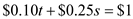Setting the Lagrange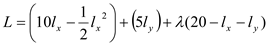Yields the first order conditions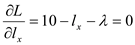....... (3)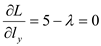...... (4) Dividing equation (3) by equation (4), we get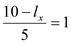So,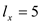Substitution of this value of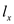into the constraintWe get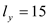The number of fish caught is then given by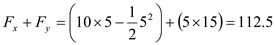c) Instead of restricting the number of people allowed to fish in lake x, a license can also be sold at price t for fishing in that lake. The price of t should be such that at the social optimum allocation ofthe following condition holds.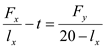So,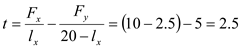d) On comparing part a) and part b) we see that the total catch of fish increases when property rights are assigned. This is because when an angler decides which lake to fish in, he considers the average catch and not the marginal catch. Though the average catch maybe positive, the marginal catch maybe negative because of externality. The angler's decision to fish in lake x decreases the catch of the anglers already fishing. He does non take that into account in his decision. However, when property rights are assigned this externality is taken into account.

a) A perfectly competitive firm is a price taker. So to maximize profits the firm will equate the price with the marginal cost. The price is given as \$10 per barrel. However, the cost is given in terms of\$1000 per well. Hence, the cost per unit would be \$1000/ q where q is the output per well. The firm would equate the MC with the price. So,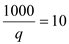As,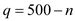, We get,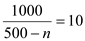So the number of wells would be equal to 400. The optimum quantity is given by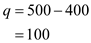There is a divergence in the private marginal cost and social marginal cost in this industry because the number of wells a firm decides to put does not only affect his quantity but reduces the quantity that can be drilled by other firms. This is because quantity of each well is negatively related to the number of well. This fact is not taken into account by the firms. b) If the oil fields are nationalized then the government will maximize total profit and not the profit of individual firms. Profit is given by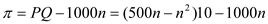The first order condition yields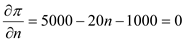So optimal number of wells is 200 Total Output is given by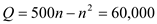Output per well is given by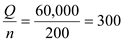c) As shown in part b) the socially optimum number of wells is equal to 200. This can be achieved through the market mechanism if appropriate property rights are put in place. This can be done through putting an annual license fee in place. Let the license be l, then the following condition should hold for n = 200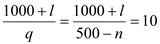The above condition shows that the cost for operating a well annually goes up from \$1000 to \$1000+ l So for optimal number of wells equal to 200,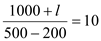We get,